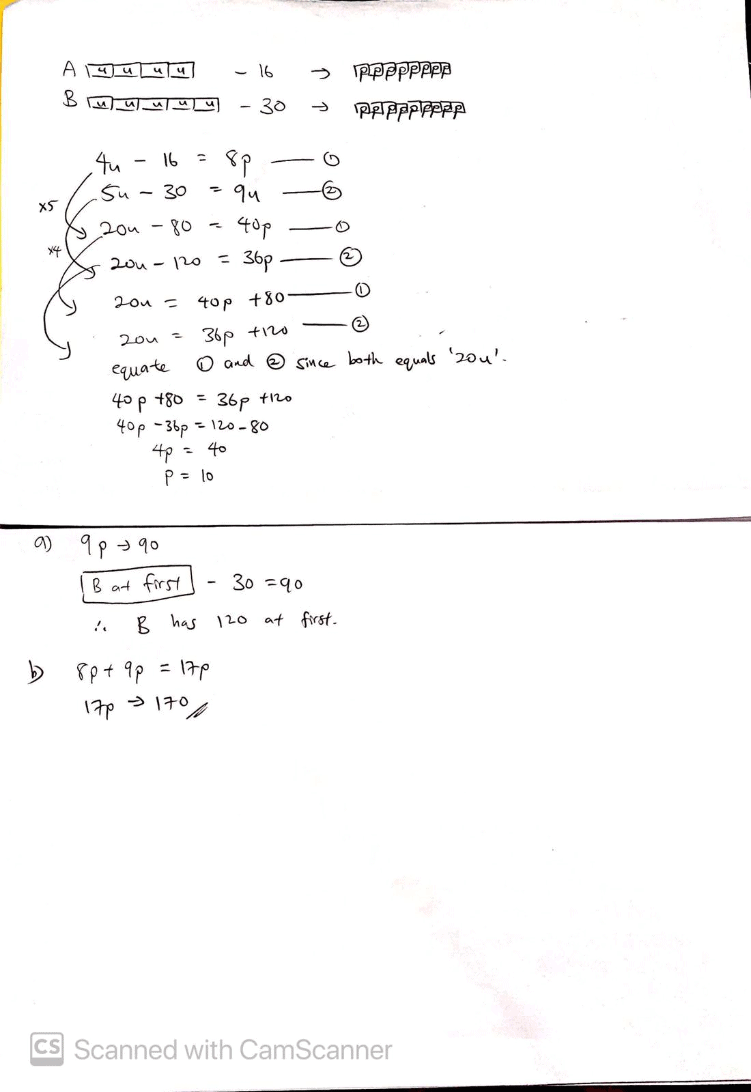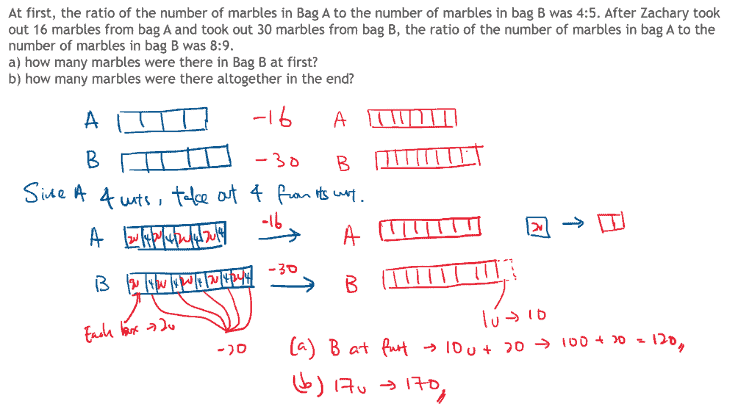# Question

At first, the ratio of the number of marbles in Bag A to the number of marbles in bag B was 4:5. After Zachary took out 16 marbles from bag A and took out 30 marbles from bag B, the ratio of the number of marbles in bag A to the number of marbles in bag B was 8:9.
a) how many marbles were there in Bag B at first?
b) how many marbles were there altogether in the end?

I came across this website. It contains so many free samples. I used them to do my college essay and got a high grade. I’ll use this platform again.

0 Replies 0 LikesThis is a concept we call “parts and units”. The name is as such because, in the above model that we draw, we include 2 types of boxes (different sizes). We call one type “unit” and another type “part”. Hence, they are also labeled “u” and “p”.

Reading the question, we understand that there are 4 units in Bag A at first, and after taking out 16, there is 8 parts left. In Bag B, there were 5 units at first, and after taking out 30, there were 9 parts left.

We write them out as 2 equations shown in the steps that are provided and we label them as ‘1’ and ‘2’. We go on to manipulate the equations ‘1’ and ‘2’ accordingly. We can see how it is solved.

We manage to find the value of ‘p’ first, which is 10.

Using the value of ‘p’ as 10, we will be able to answer the questions part a) and b).

This method used should be one of the most comprehensive model methods available. It is based-after a secondary school mathematics concept called “simultaneous equations’ but have been simplified to cater to the younger primary school students as models are used to aid the students visually.

Do PM me if you need clarification. Happy to help. (:

www.futureachievers.com.sg

0 Replies 1 LikeThis requires your child to have both “Before and After” concept and ratio concept. After drawing the models you realise that there is a missing unit which equates to 10.

So the key here is realising that 16 can be divided into 4 units and you can take 4 out of each unit from A.

This is what we do in Enso Education, focusing on core concepts and keeping complicated word problems simple with model drawing and restating the key concepts involved. As the questions may change, the key concepts remain unchanged.